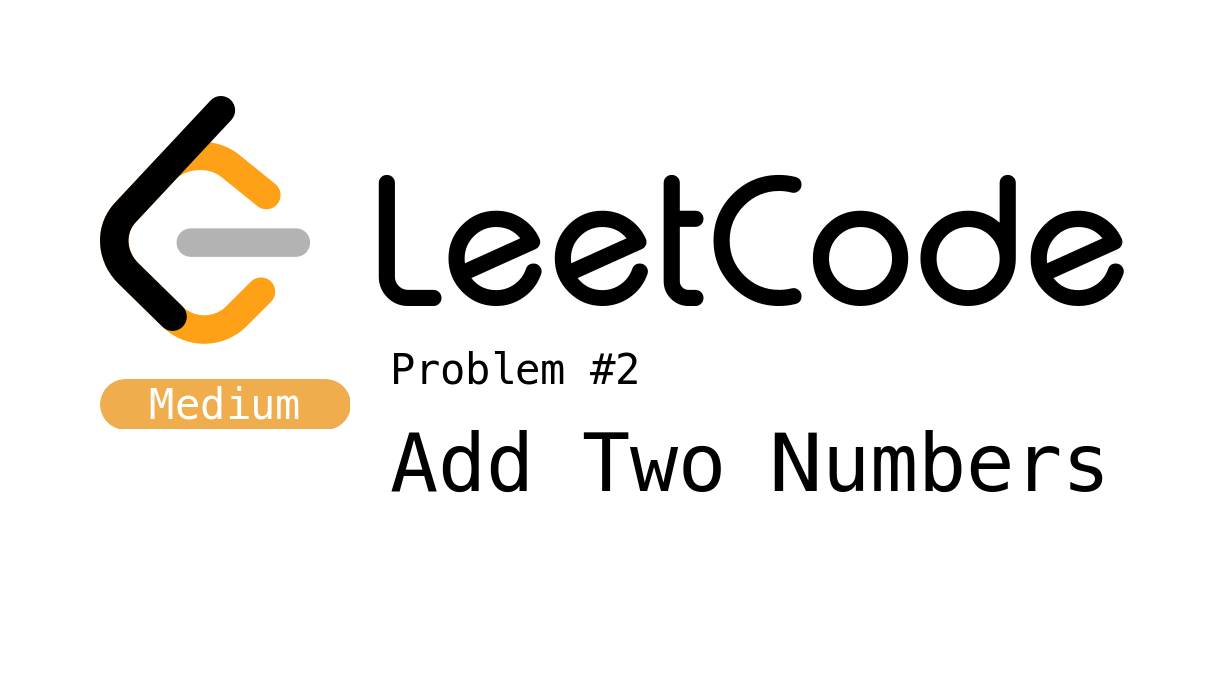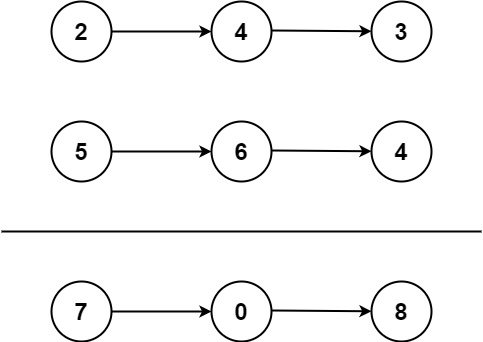# LeetCode Problem 2 - Add Two NumbersIn this post we’ll be talking about solving the second LeetCode problem. You can find my code for all my LeetCode submissions at GitHub.

LeetCode is a site that provides programming problems for practice. A lot of developers use it to hone their skills or to practice for upcoming interviews. The questions available are used by large tech companies as interview questions, so it’s a good place to start and the difficulty of the questions being Easy, Medium or Hard.

While the solutions for these problems are available directly from the site, they are directly to the point and can be a bit technical. I’d like to use these types of posts to explain the thought process to get to the solution, and how to think about and test for edge cases. I’ll be using C# in dotnet to create my solution. Download Visual Studio for free to create your own.

### The Problem

You are given two non-empty linked lists representing two non-negative integers.

The digits are stored in reverse order, and each of their nodes contains a single digit.

Add the two numbers and return the sum as a linked list.

You may assume the two numbers do not contain any leading zero, except the number 0 itself.


The link to the problem can be found here.

It is important to catch the fact that the list is the digits of the number in reverse order.

Here is an example:In this case, the input would be List1 = [2,4,3] which represents 342 and List2 = [5,6,4] which represents 465.

The output for this example should be [7,0,8] because 342 + 465 = 807.

Another example could be something like List1 = [0,0,0,1], representing 1000 and List2 =  representing 1.

This would result in the output 1001.

The problem provides you with a class definition for a linked list.

public class ListNode
{
public int val;
public ListNode next;

public ListNode(int val = 0, ListNode next = null)
{
this.val = val;
this.next = next;
}

}


A linked list is made up of nodes. Each node contains a piece of information, which is its value or data, and then a pointer to another node. These nodes pointing to other nodes, makes a chain. If the next pointer in the chain is nothing, or null, then we know that we are at the end of the list and should stop.

A linked list is similar to an array in that it stores a list of data, but it can’t be randomly accessed. That is to say, you can’t just select the 5th item in a linked list chain without first going through the first 4 items.

Also, arrays are stored in contiguous memory, which means the data in the array is all lined up nicely so it can be randomly accessed. This means that if you don’t have enough memory in one chunk to allocate to your array, it will fail. The advantage of the linked list is that the data can be anywhere and each node points to where the next piece of data is.

Side note, there is such a thing as a doubly linked list that has both next and previous pointers.

### The Test Cases

I am a huge believer in automated testing. Unit testing, integration testing, end to end testing, they are all needed to make sure you have confidence in the quality of your code. Your product is only as good as your tests. That said, of course we’re going to unit test our solution before submission!

This is a particularly fun problem because there are some interesting edge cases to think about. The hard part is, we need to setup some helping code to test things.

Below you’ll see two helper functions I wrote to facilitate testing. The first builds a list for us from an array. The second takes a list, and outputs the array value.

public ListNode BuildList(int[] input,int index=0)
{
return index+1 < input.Length ? new ListNode(input[index], BuildList(input, ++index)) : new ListNode(input[index]);
}

public int[] Output(ListNode n)
{
var res = new List<int>();
var node = n;
while (node != null)
{
node = node.next;
}

return res.ToArray();
}


With these functions, we can now input an array of numbers to get our list, then take the resulting list of our solution and check it’s output to make sure it’s correct. But, we need to make sure that part is working correctly as well, so here is a test checking those functions.

[Test]
public void ShouldBuildListNodeFromIntArray()
{

var input = new[] {2, 4, 3};
var node = sut.BuildList(input);
input.ShouldBe(sut.Output(node));

input = new[] {5, 6, 4};
node = sut.BuildList(input);
input.ShouldBe(sut.Output(node));

input = new[] {9, 9, 9};
node = sut.BuildList(input);
input.ShouldBe(sut.Output(node));

input = new[] {0, 0, 0, 1};
node = sut.BuildList(input);
input.ShouldBe(sut.Output(node));

input = new[] {7, 0, 8};
node = sut.BuildList(input);
input.ShouldBe(sut.Output(node));

input = new[] {1};
node = sut.BuildList(input);
input.ShouldBe(sut.Output(node));

input = new[] {0};
node = sut.BuildList(input);
input.ShouldBe(sut.Output(node));

}


Since the two helper functions do the opposite of each other, we can test them against each other to make sure we get the original input array as a result.

Now that we have our helper functions setup and tested, lets think about this problem a bit more and some edge cases surrounding it.

• The lists are different lengths. In the example above, we used [0,0,0,1] and .
• The addition requires a carry. What if we add [9,9,9,9] and 
• The zero case. What if we add  to a list? What if we add  to ?
• The lists are the same length, but add up to more than 10. Think about adding  and 

Now that we have some edge cases to think about, lets setup those kinds of tests

[Test]
{

var l1 = sut.BuildList(new[] {2, 4, 3});
var l2 = sut.BuildList(new[] {5,6,4});
sut.Output(res).ShouldBe(new[] {7, 0, 8});

l1 = sut.BuildList(new[] {0});
l2 = sut.BuildList(new[] {0});
sut.Output(res).ShouldBe(new[] {0});

l1 = sut.BuildList(new[] {9,9,9});
l2 = sut.BuildList(new[] {9});
sut.Output(res).ShouldBe(new[] {8,0,0,1});

l1 = sut.BuildList(new[] {9,9,9,9,9,9,9});
l2 = sut.BuildList(new[] {9,9,9,9});
sut.Output(res).ShouldBe(new[] {8,9,9,9,0,0,0,1});

}


### The Solution

For this solution, I chose to go with a recursive function. A recursive function is a function that calls itself until it reaches a section of code that causes it to stop calling itself. Be careful though, if there is no such case, it can cause a stack overflow or an infinite loop.

Here is my solution:

public ListNode AddTwoNumbers(ListNode l1, ListNode l2)
{
return SumLists(l1, l2, 0);
}

public ListNode SumLists(ListNode l1, ListNode l2, int carry)
{
if (l1 == null && l2 == null && carry == 0)
{
return null;
}
var val1 = l1 == null ? 0 : l1.val;
var val2 = l2 == null ? 0 : l2.val;

var sum = val1 + val2 + carry;

carry = sum >= 10 ? 1 : 0;
if (carry == 1)
{
sum -= 10;
}

return new ListNode(sum, SumLists(l1?.next, l2?.next, carry));
}


As you can see, the AddTwoNumbers function just passes in the two lists we’re working with and a carry of 0, because we haven’t done any calculations yet, so we have nothing left over.

The first step in the SumLists function is to setup our exit case. If there is nothing left to add in our lists and the carry is 0, we’re done and can return null.

Next, we’ll add the values of our current list nodes together. If they are null, meaning they don’t exist, we’ll just add 0 instead. But, we can’t forget anything that carried over from a previous calculation, so we add that in as well. On our first pass, this will always be +0.

Now we need to check if our current calculation is bigger than 9 causing a two digit number, those aren’t allowed as data in our nodes. If our sum is greater than or equal to 10, we know we need to carry a 1 over to the next calculation, and if we do carry, we need to subtract that 10 from the current sum.

Finally, we have the recursive part. This function is going to return a new ListNode. It’s value will be our sum, and it’s next node will be the calculation of the next nodes in each list, along with any carry we calculated in the current pass. Notice the ? in l1?.next and l2?.next, that makes sure to put a null in that place if l1 or l2 are themselves null. This makes sure that if one list is larger than the other, we can carry on until there is no more reason to do so.

### Conclusion

I enjoyed this problem very much. Thinking recursively is an interesting exercise, and can make for very consice and clean code. If you found some better edge cases, or a more interesting way to solve this problem let me know! As extra credit, see if you can make the function tail-recursive.

You can find all my LeetCode submissions at GitHub. I hope this was helpful and look forward to more problem solutions in the future.